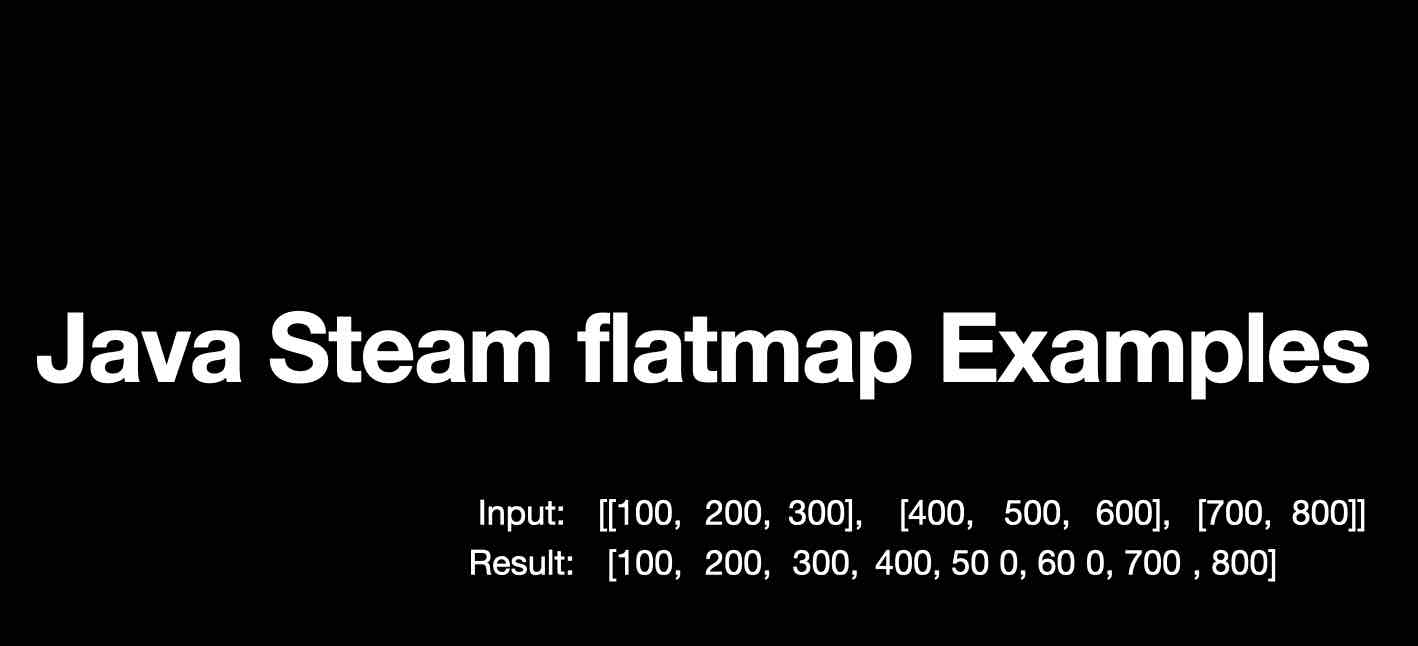# Java Stream flatmap() Examples

In Java flatmap() is a function in the Stream class that can be used to flatten a Stream of Collections into a single Stream of Objects.

✏️Note: flatmap() is available only since Java 1.8

Example 1: Concat a 2-Dimensional Integer Array into a single 1-Dimentaional Array
``````import java.util.Arrays;
import java.util.stream.Stream;

public class JavaFlatMapExample {

public static void main(String[] args) {

Integer[][] array2D = new Integer[][]{{100, 200,300}, {400,500,600}, {700, 800}};
System.out.println(Arrays.deepToString(array2D));
Stream<Integer[]> streamOf2DArray = Arrays.stream(array2D);
Integer[] array1D = streamOf2DArray.flatMap(Stream::of).toArray(Integer[]::new);
System.out.println(Arrays.toString(array1D));
}
}``````
Output:

Input: [[100, 200, 300], [400, 500, 600], [700, 800]]
Result: [100, 200, 300, 400, 500, 600, 700, 800]

Example 2: Concat a 2-Dimensional String Array into a single 1-Dimentaional Array
``````    public static void main(String[] args) {

String[][] array2D = new String[][]{{"one", "two"}, {"three","four","five"}, {"six", "seven","eight","nine","ten"}};
System.out.println(Arrays.deepToString(array2D));
Stream<String[]> streamOf2DArray = Arrays.stream(array2D);
String[] array1D = streamOf2DArray.flatMap(Stream::of).toArray(String[]::new);
System.out.println(Arrays.toString(array1D));
}``````
Output:

Input: [[one, two], [three, four, five], [six, seven, eight, nine, ten]]
Result: [one, two, three, four, five, six, seven, eight, nine, ten]-

Have Questions? Post them here!